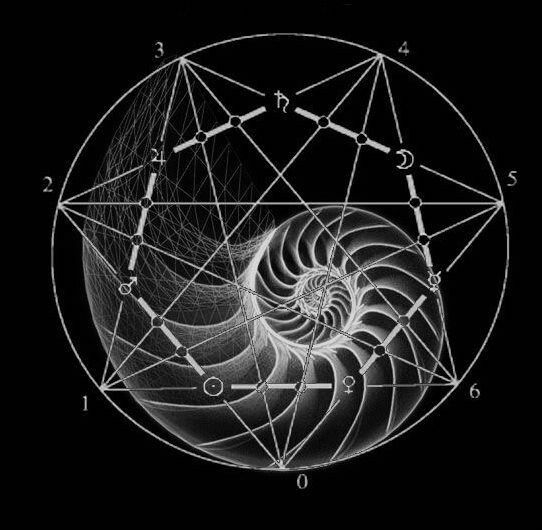top of page
Search

# 7 and the Fibonacci spiral part 1The 7 pointed star encodes the Fibonacci spiral and 7 creates the enneagram and the 64 tetrahedron grid and is the seed of life and 7 is in the tetragrammaton which encodes 137 which is encoded in 432

7 is used in:

1/7=0.142857=enneagram

1+2+3+4+5+6+7=28=(64 tetrahedron grid)

Tetragrammaton=((Π^7)/(Π7))=137.3

(13×(33.3))÷Π=137.7

(13×(33.3))=432.9